# Excel Array Formulas: What Is It, And How to Use It

Jul 01, 2023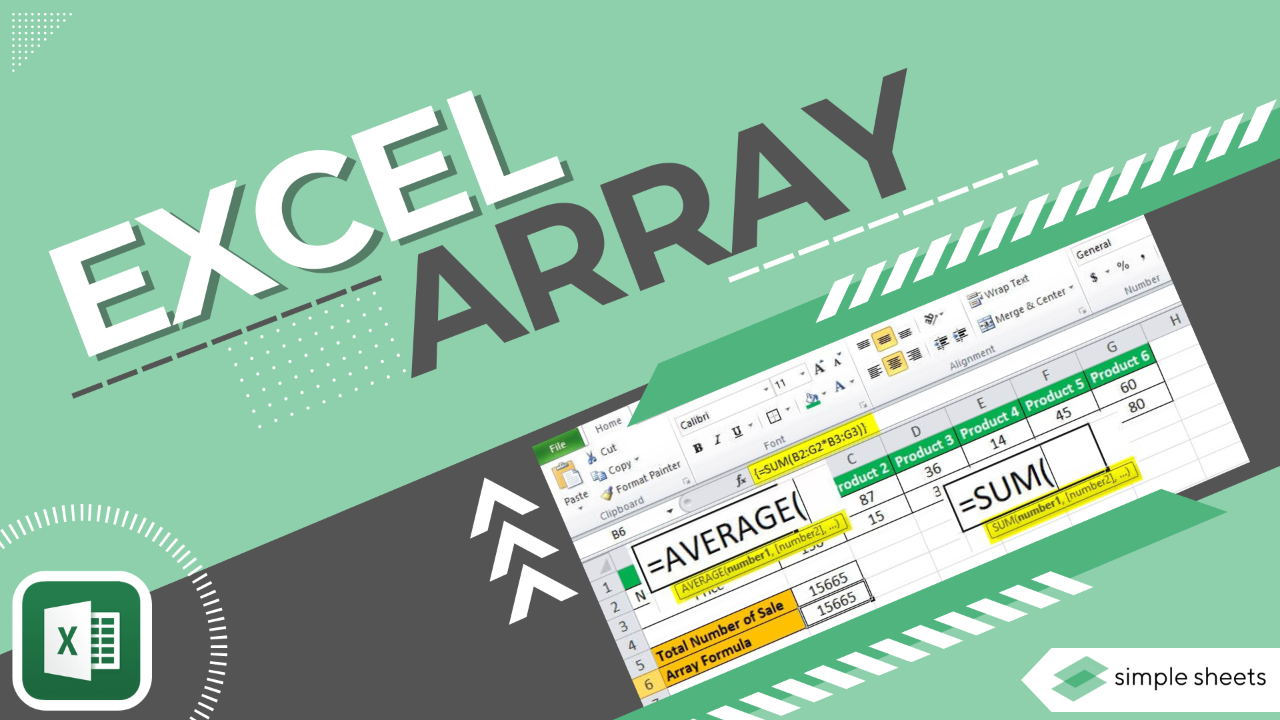Trying to figure out array formulas in Microsoft Excel?

Look no further! Excel array formulas are an incredibly powerful and useful tool that can help you analyze data, perform calculations, and accomplish complex tasks quickly.

In this blog post, we will explore Excel array formulas, specifically the available types, how they work, and the best practices for using them.

Read on as we cover the following:

• What Are Array Formulas?

• Why Use Array Formulas in an Excel Worksheet?

• How to Create and Use an Excel Array Formula

• Editing and Deleting an Excel Arrays Formula

• Types of Array Formulas

• Using Arrays to Move Rows and Columns

• Frequently Asked Questions on an Excel Array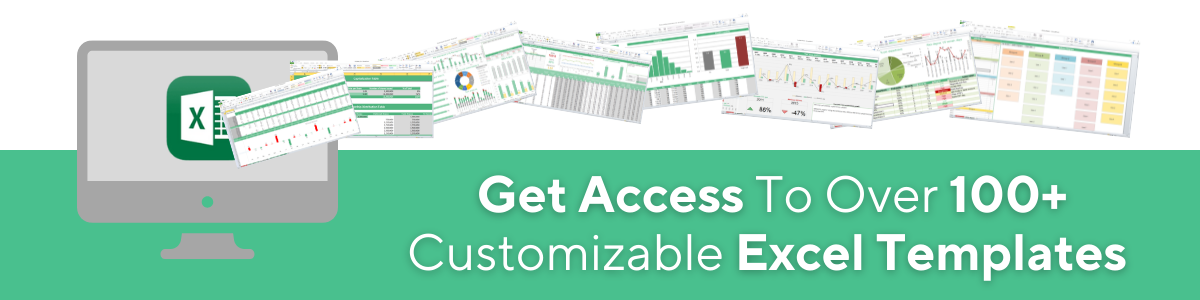## What Are Array Formulas?

The array formula in Excel is almost the same as regular formulas.

However, one characteristic distinguishing them from regular formulas is that they can process several values ​​rather than just one in a single operation.

In spreadsheet programs, an array is a range or series of related data values ​​typically stored in adjacent cells on a worksheet.

The Excel array formula evaluates all the individual values ​​in an array. It performs multiple arithmetic operations according to the conditions expressed in the formula.

Array formulas are useful for Excel because they can do many calculations simultaneously. Moreover, they give back more than one answer at the same time.

So, the results that an array formula returns are also an array.

## Why Use Array Formulas in Excel Worksheet?

Excel array formulas are useful in complex calculations, especially since they simplify challenging tasks. With a single formula, you can replace hundreds of other formulas.

Array formulas can effectively achieve the following functions:

### Sumnumbers:

Array formulas sum numbers that meet specified conditions. For example, you can sum the numeric value "X" and the most significant or minor numeric value in a range.

### Sum every other row:

Excel array formulas add numbers in every row or column containing the numeric value X, given above as an example.

### Count characters:

Array formulas can count all or some characters in a specified range.

## How to Create and Use an Excel Array Formulas

We'll use the following table as sample data to display how to create and use an array formula.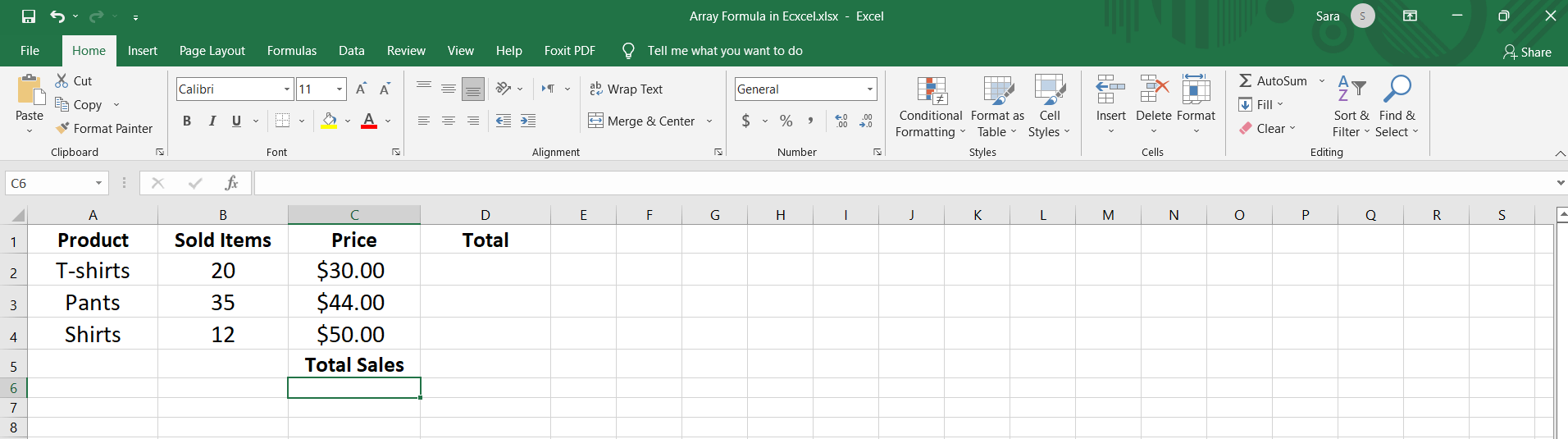This example shows the number of items sold for each product during a given period.

In addition to the price, we also need to calculate the sum of sales of all products:

Many Excel users may choose to compute the total sales by adding a column to find the sales value of each product. Then, they add them up to get the total sales.

But, you can shorten these steps by writing a single formula.

1. Select a cell which in cell D5, then type the equal sign and the SUM function.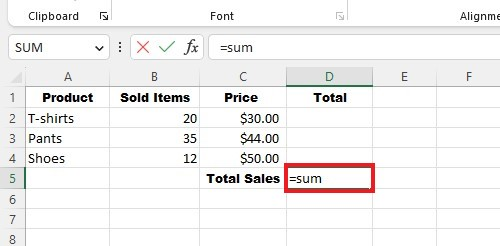1. Next, we open brackets and specify the number of sold items for all products.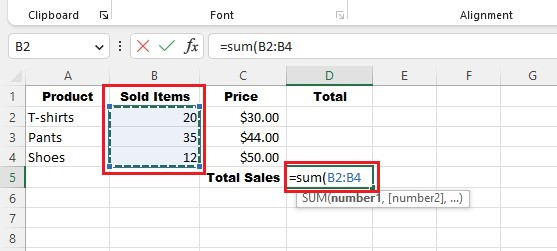1. Finally, we set the item price for each product (i.e., range of cells C2:C4) and close the bracket, so the formula is as follows: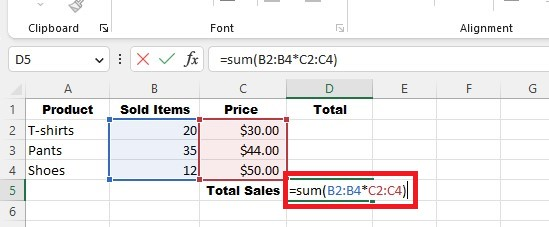1. Press the "Ctrl + Shift + Enter" instead of the Enter key only to get the result:Notice that curly opening and closing braces (curly brackets) appear in the formula bar at the beginning and end of the formula automatically. These two curly brackets indicate the array formula.

Do not attempt to create an array formula by manually entering curly braces in any empty cell. You will never get the same result because Excel will treat the input as text and not as an Excel formula.

The formula will work like this:

Each value in the 'Sold Items' column will be multiple calculations by its 'Price' column, and then the answers are summed to get the total sales.

The array formula can also return multiple results if you want various values.

For example, if we want to find sales for each product separately, we select the cell range D2:D4 in the Sales column and write the following formula: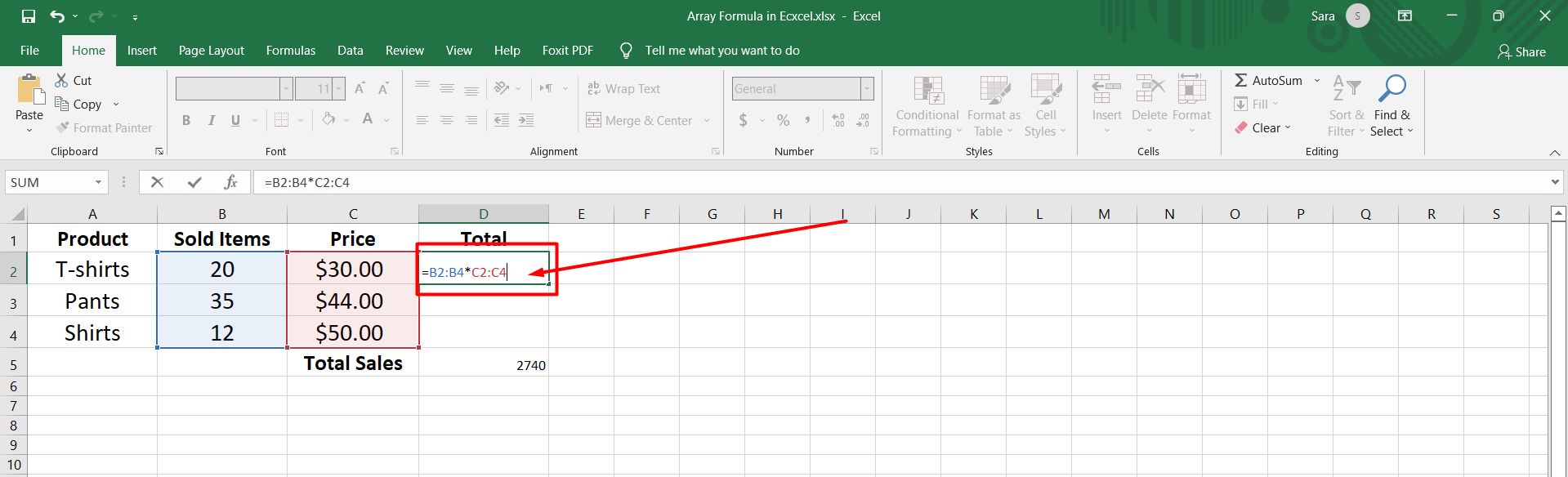Note:

And remember to press Ctrl+Shift+Enter to return the results. Because pressing Enter will only return one result in cell D2: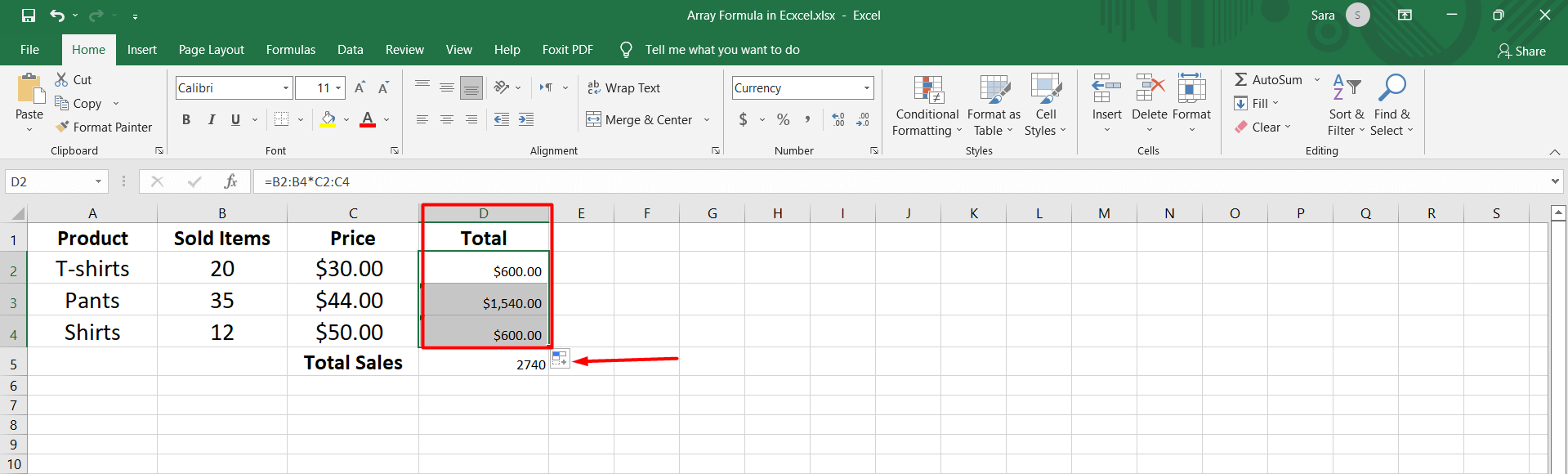In the array formula, you can use different functions like AVERAGE, MAX, MIN, and many more built-in Excel functions.

For example, to calculate average sales for the same table, we write the following formula: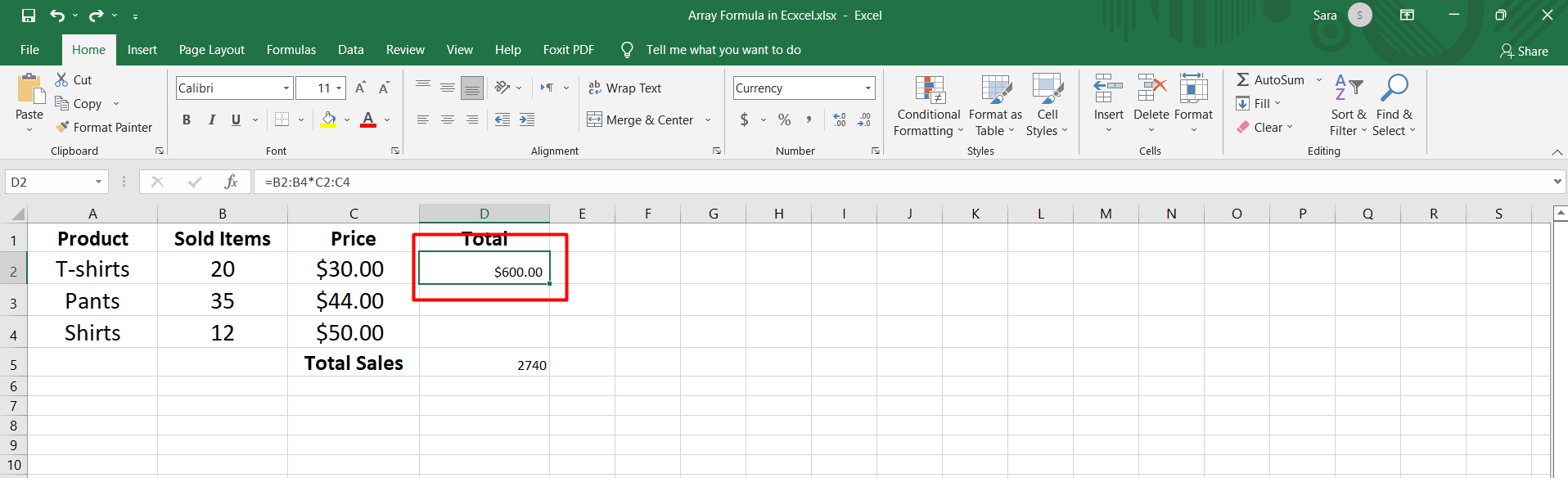Then, press Ctrl+Shift+Enter to find the result.

The formula will multiply the number of sold items by their price. It will add up all the sales and divide it by the number of products.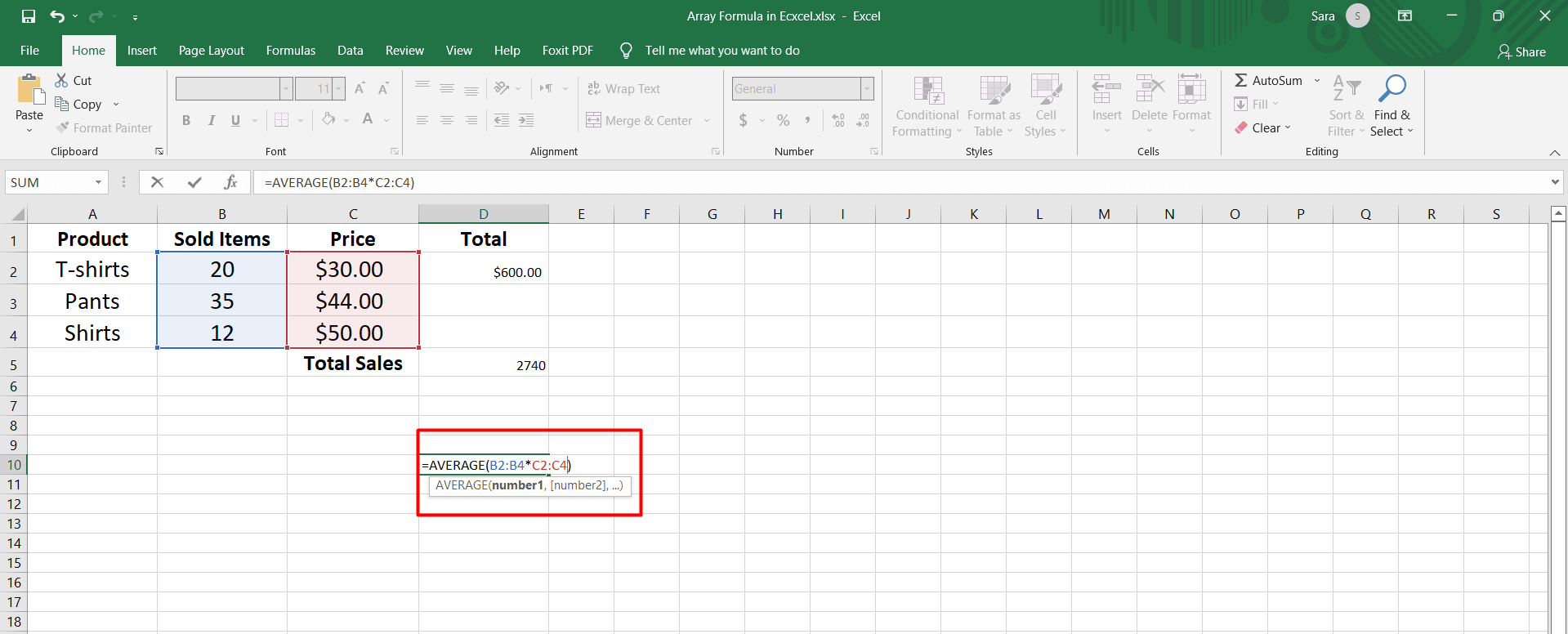If you want to find the product with the highest sales, select the appropriate range. Then, write the following formula: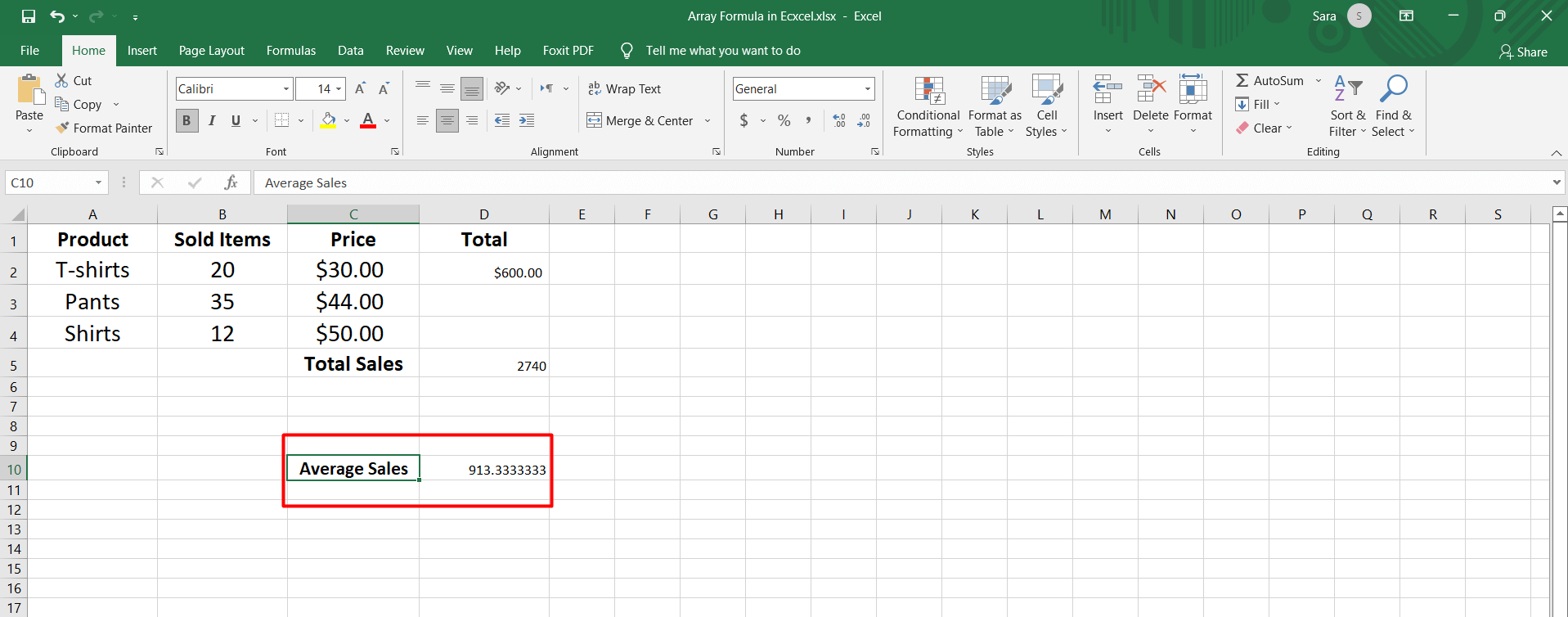Press Ctrl+Shift+Enter to calculate the sales of each product and then return the highest value of sales in the output cell: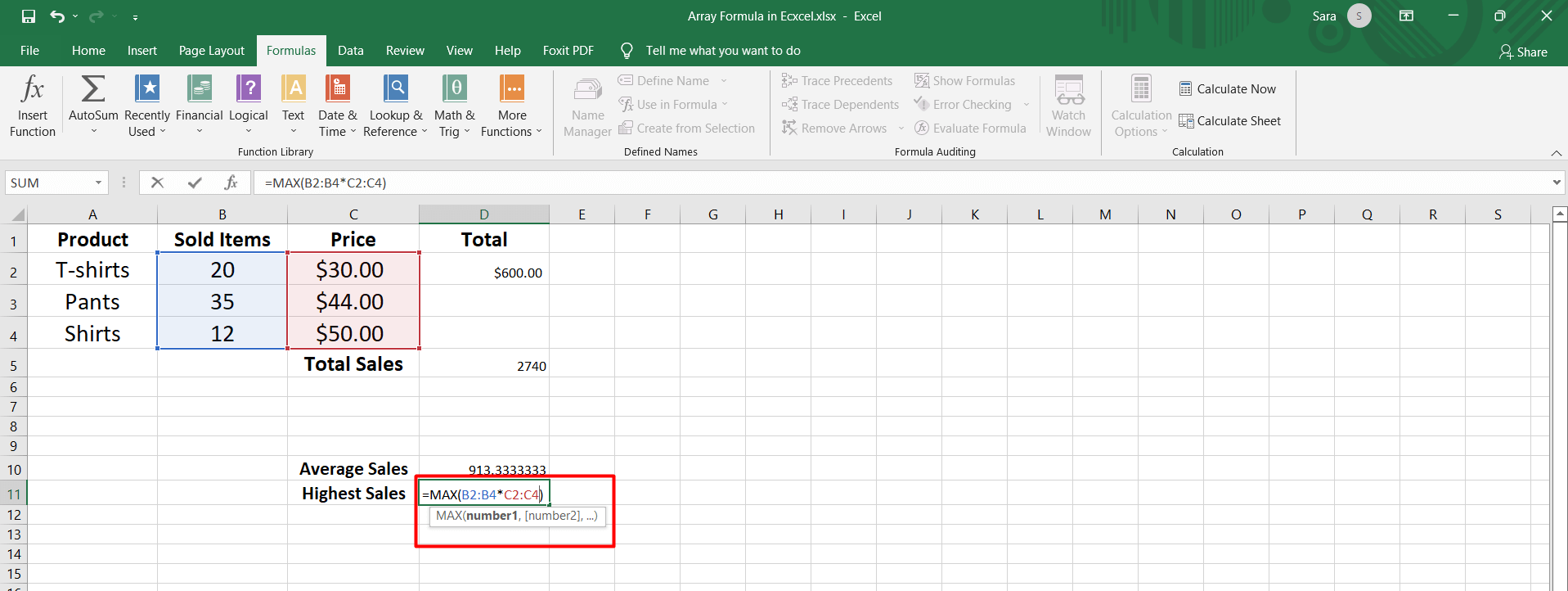## Editing and Deleting an Excel Array Formula

Editing and deleting array formulas requires special steps, mainly if they apply to multiple cells rather than a single cell.

To edit the array formula in a single cell, select the cell.

Then, press F2 to turn on edit mode. Modify the syntax as required, e.g., shrink or extend the range.

Let's say we want to modify the sales group formula because we added a fifth product.

So, we will edit the formula and select the ranges B2:B5 and C2:C5 instead of B2:B5 and C2:C5, then press Ctrl+Shift+Enter: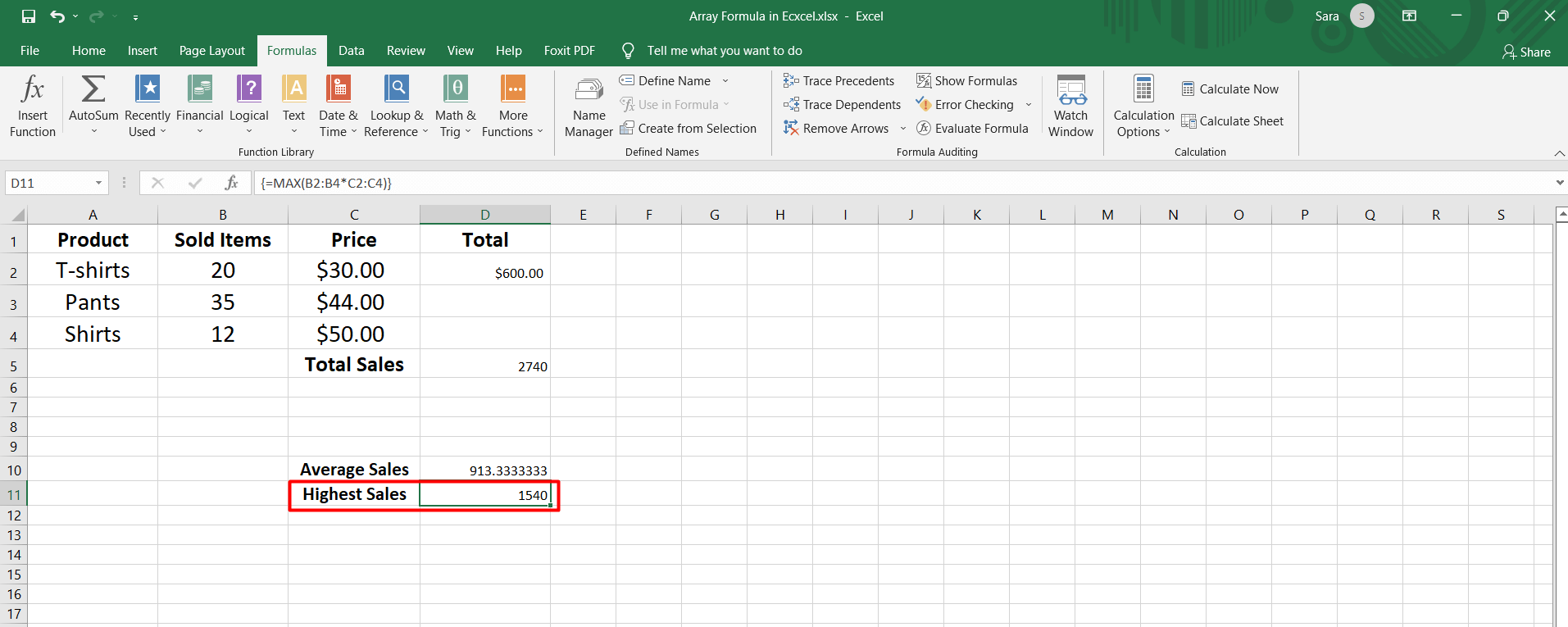You will find this result in the image below.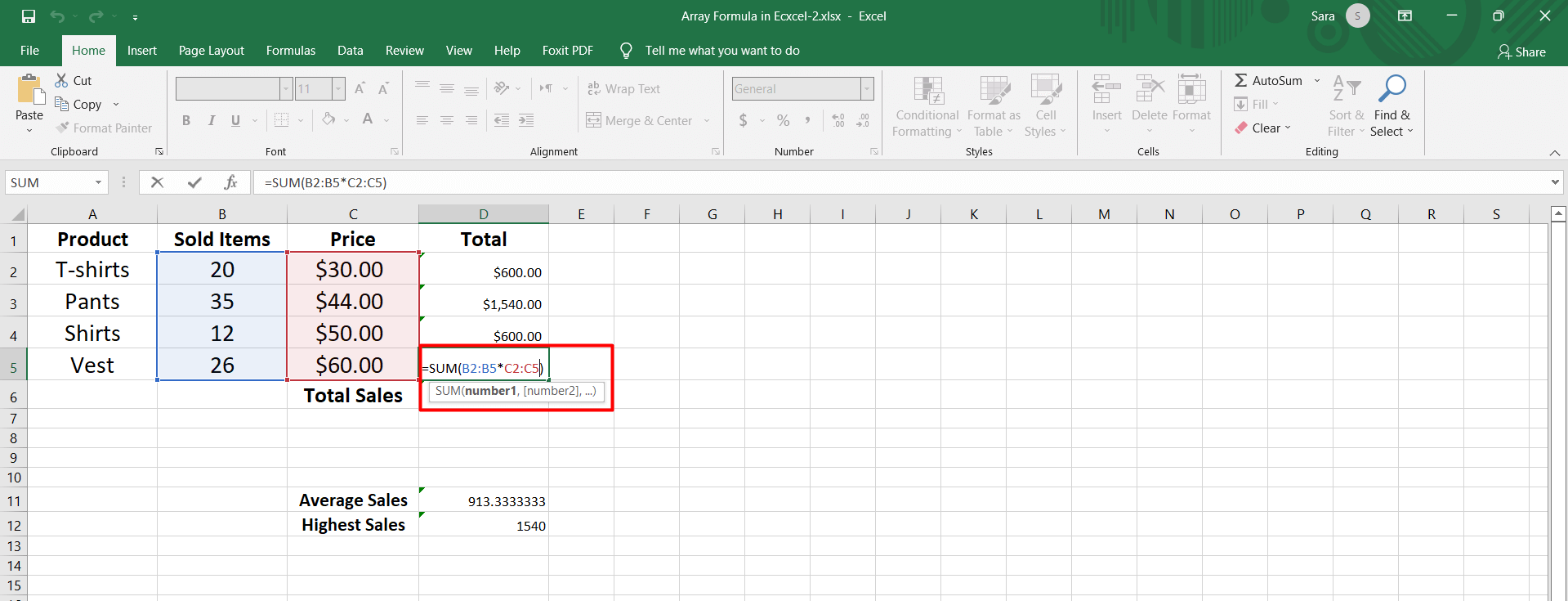If you apply the array formula to the cell range, you will find the sales value of each product separately.

In this case, you must select the entire cell range, press F2, make the desired modification, and then press Ctrl+Shift+Enter: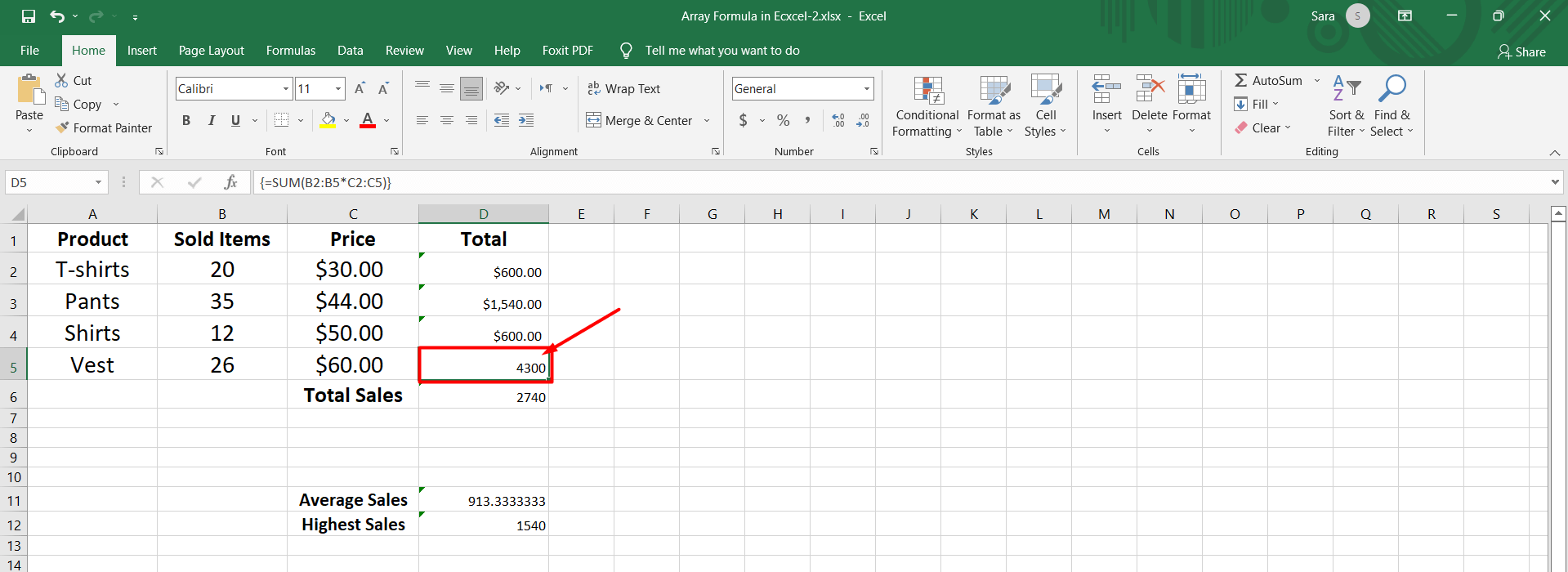You can't delete an array formula if a single cell belongs to a range of cells.

Try doing this by selecting the cell and pressing the Delete key.

Excel will warn you that it is not possible to change part of the array through a small dialog box: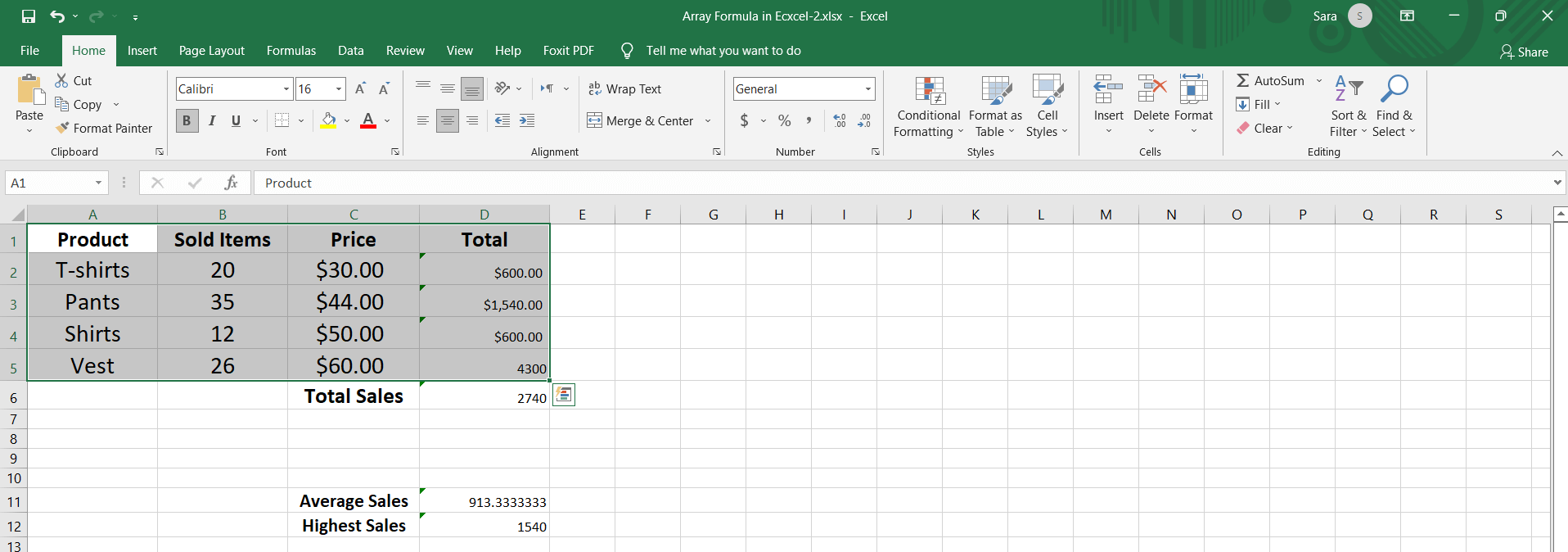If you want to delete a cell of an array, you must remove the entire array and rewrite the array formula again.

The same goes for the array's moving (cutting and pasting) part; you get the same alert.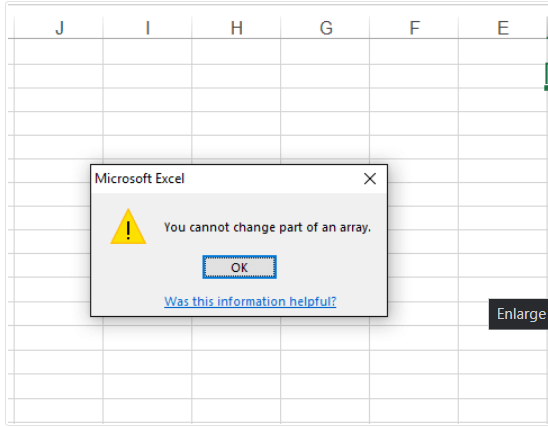But you can cut the entire array and paste it somewhere else.

The cell references do not change when moving but will remain the same.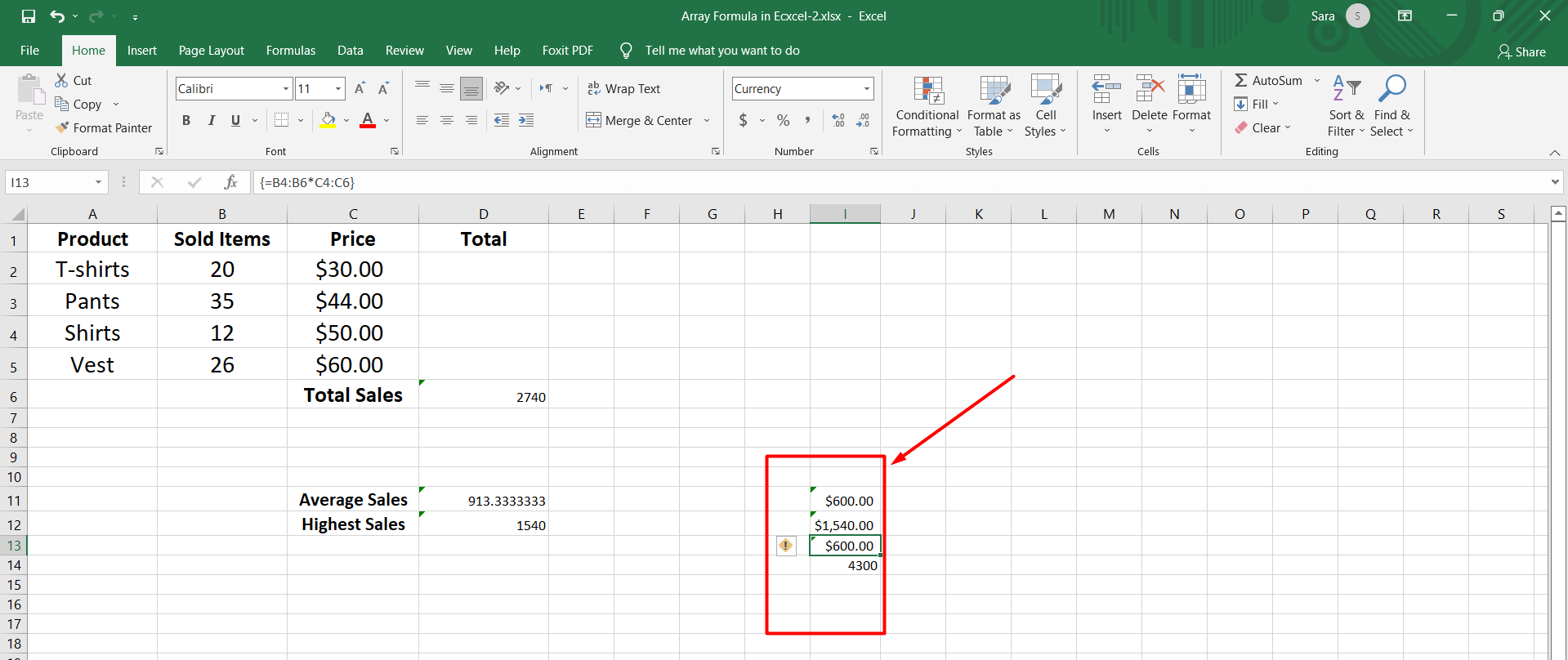## Types of Array Formulas

There are two types of array formulas:

• Single-cell array formulas that perform multiple calculations in one cell.

• Multi-cell array formulas that are in more than one cell.

### Single-cell Array Formula

This type of array formula uses a function, such as SUM, RATE, or COUNT, to combine the output of a multi-cell array formula into a single value in a single cell.

An example of a single-cell array formula would be: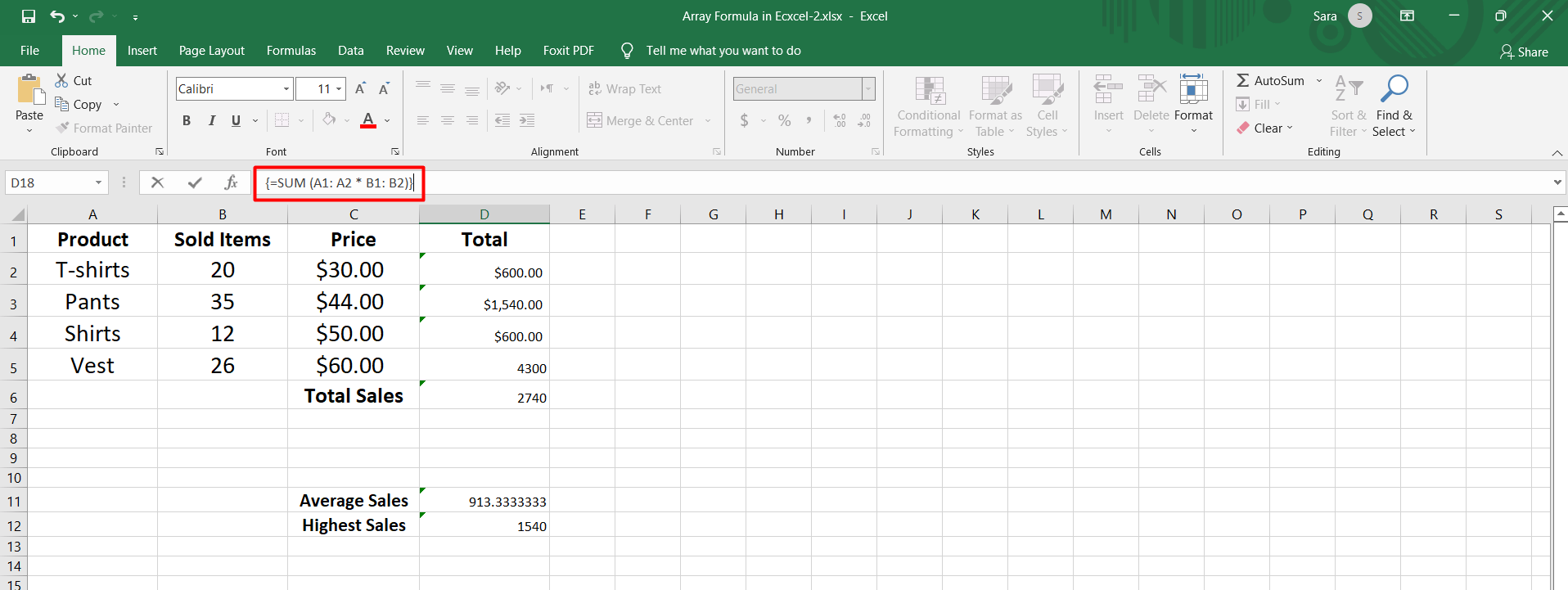This formula adds together the A2 B2 and A3 B3 products, and then the array formula returns, resulting in single-cell array formulas in the worksheet.

Otherwise, you can write the formula like this, and it will give you a similar answer as single-cell array formulas: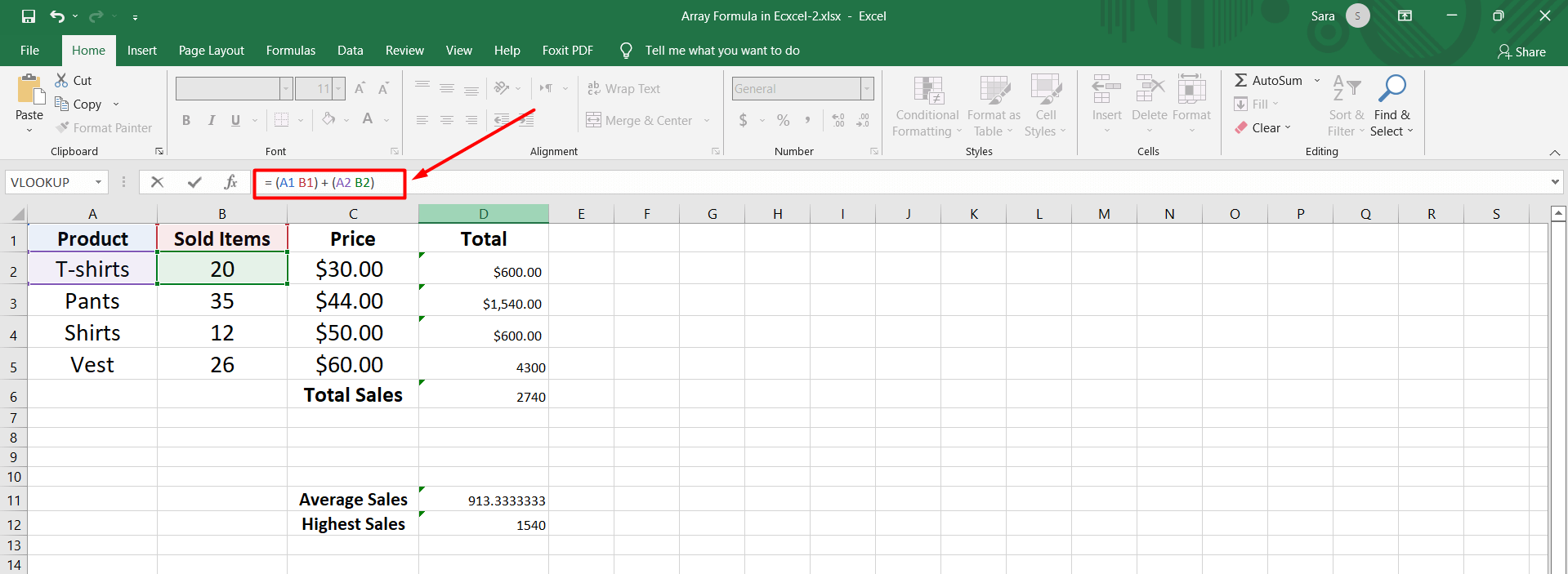### Multi-Cell Array Formula in Excel

You can locate a multi-cell formula in the muti-cell array formulas that display an array as a result.

In other words, the same formula exists in the multi-cell formula and returns different answers in each cell.

Each copy, or fragment, of the array formula, performs the same calculation in every cell it inhabits, but each copy of the formula uses different data in its multiple calculations.

So, each instance produces different results. An example of a multi-cell array formula might look like this: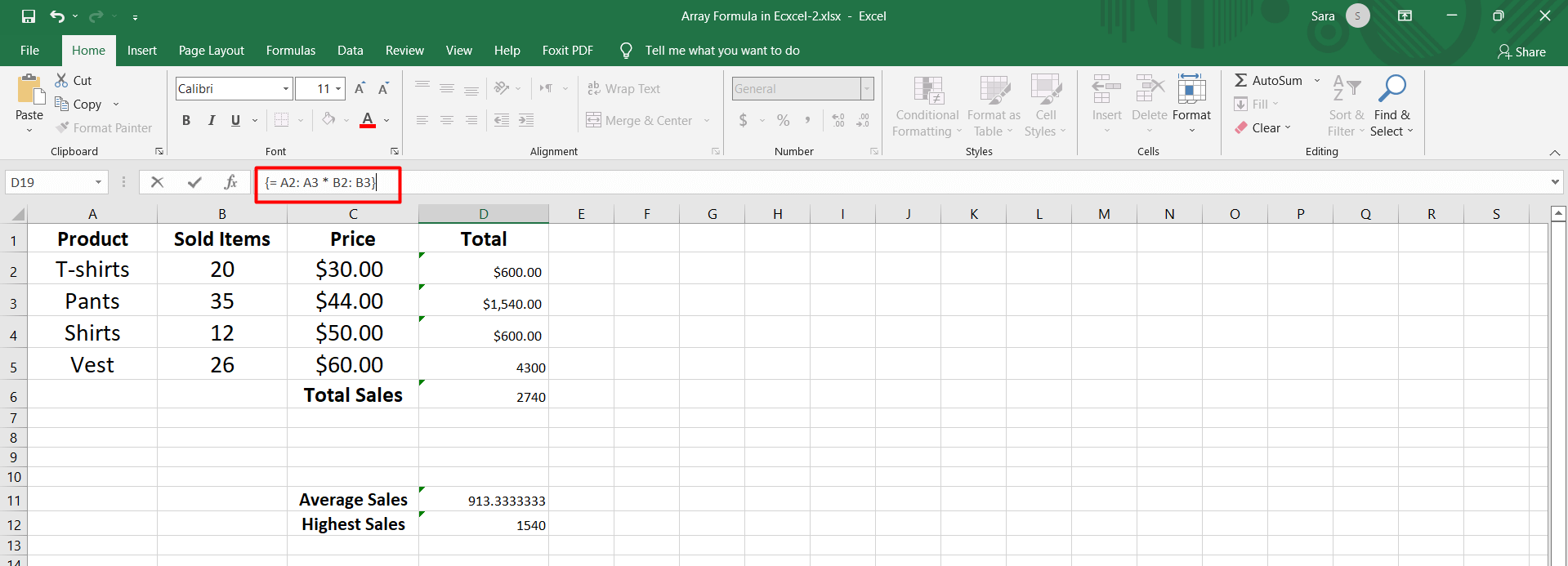If the above example is in cells E2 and E3 of the worksheet, the results will be as follows:

• The product of A2 and B2 is in cell E2.

• The product of A3 and B3 is in cell E3.

## Using Arrays to Move Rows and Columns

The TRANSPOSE function can switch data orientation from row to column or column to row.

The TRANSPOSE function is one of the few in Excel that you must use as an array formula.

### Examples of Array Formulas in Excel:

Excel has many functions, such as SUM, RATE, and COUNT.

You can use these functions in array formulas in Excel.

Some functions, such as the transposition of a function, must always be entered as an array formula to function correctly.

The utility of many functions, such as INDEX and MATCH or MAX and IF, can be extended by using them together in an array formula.

### Data cell count

To count cells that match one of the multiple conditions in an array formula, use the SUM and IF functions.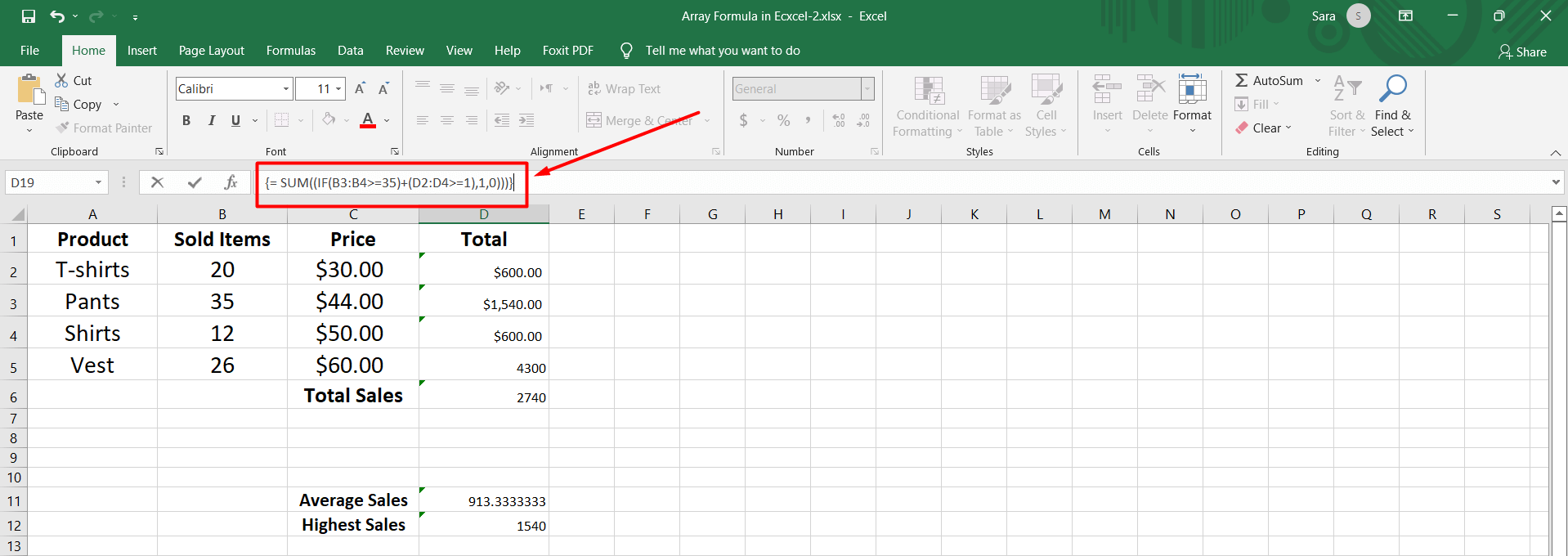The COUNTIFS function has a different approach, which only performs multiple calculations that must meet the specified conditions.

### Finding the largest positive or negative number

The MAX function in Excel can help you find the biggest number in a list. You can look for the biggest number based on different criteria or values.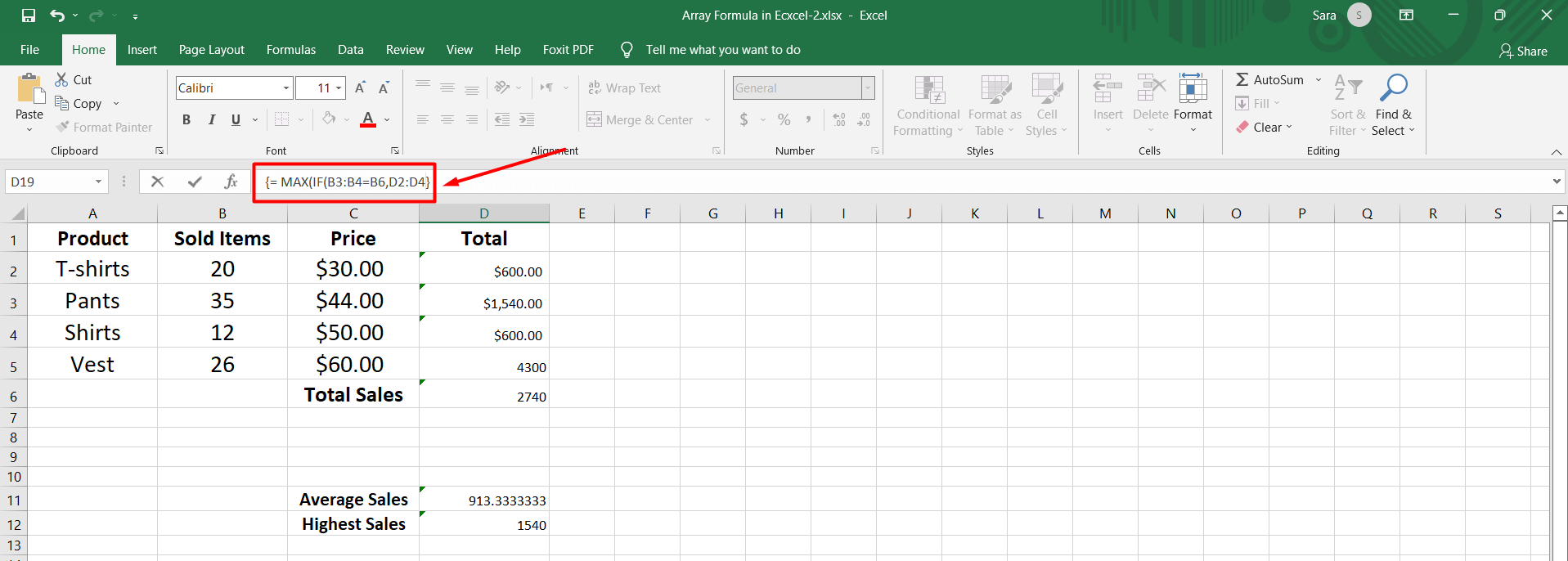### Finding the median values

The MEDIAN function in Excel finds the median value of a list of data by merging it with the IF function in an array formula.

You can find the mean value for diverse sets of related data.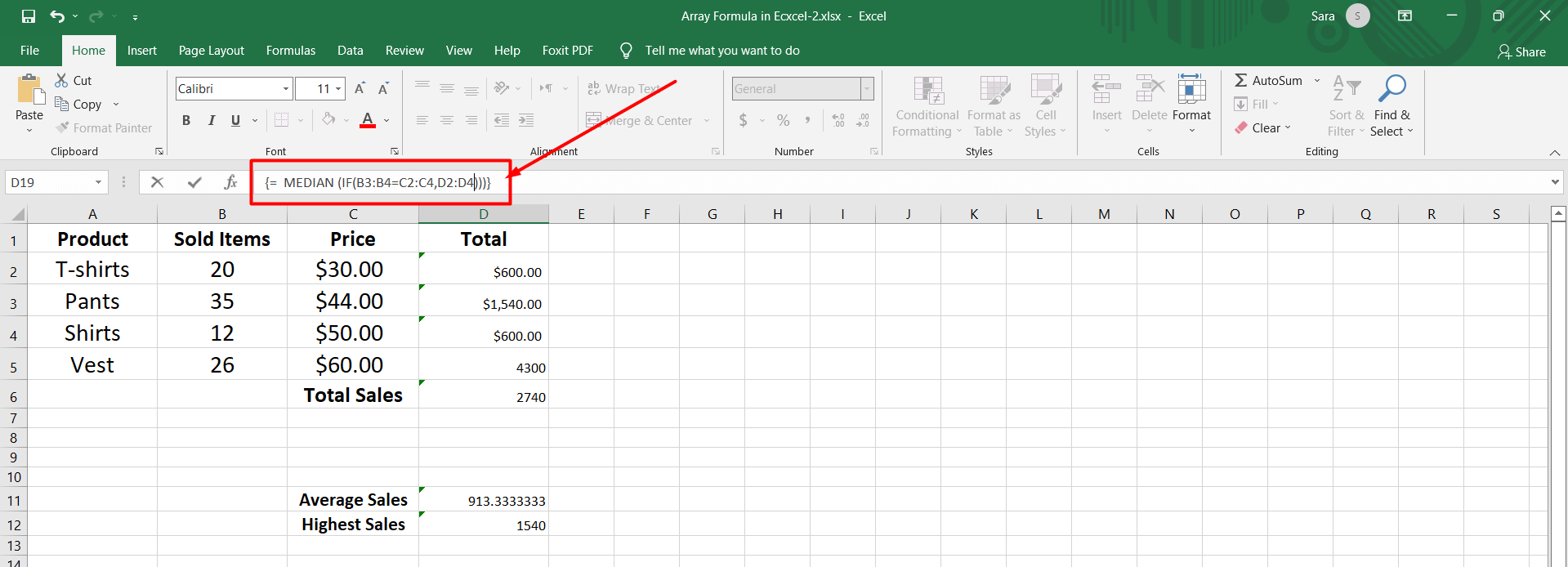## Final Thoughts on Excel Array

Arrays in Excel are powerful tools that allow users to work with multiple values or elements simultaneously.

They provide a convenient way to perform complex calculations, manipulate data, and automate tasks efficiently.

Visit Simple Sheets for more easy-to-follow guides and examples, and remember to read the related articles section of this blog post.

For the most straightforward Excel video tutorials, subscribe to Simple Sheets on YouTube!## Frequently Asked Questions on Excel Array

### Are array formulas supported in all versions of Excel?

Excel featured array formulas for a long time, including older versions such as Excel 2003 and later.

### How can I handle errors in array formulas?

You can use functions like IFERROR, IFNA, and ISERROR to check for errors within array formulas and return specific values or messages. These functions help prevent errors from propagating throughout the array calculation.

### Can I combine array formulas with other Excel functions?

You can combine array formulas with various Excel functions to perform advanced calculations. For example, you can use array formulas with functions like SUM, AVERAGE, MAX, MIN, IF, INDEX, MATCH, etc.## Related Articles

Learn How to Make a Graph in Excel With These Simple Steps

Beginners Guide: How To Change Cell Styles In Excel

How to Add a Trendline in Excel Online

#### Want to Make Excel Work for You? Try out 5 Amazing Excel Templates & 5 Unique Lessons

We hate SPAM. We will never sell your information, for any reason.